# Stock trend prediction with Machine Learning (random forests)¶

This notebook is based on blog post from quantisti.com https://blog.quantinsti.com/decision-tree/.

The article was trying to predict stock price one day ahead using decision tree algorithm and stock technical indicators.

Here we will expand on that aticle by:

• getting data from Yahoo! Finance API
• using scikit random forest algorithm
• not predicting price one day ahead, but predicting uptrend many days ahead
• using these technical indicators - Paraboloc SAR, RSI, Bollinger Bands and series of Exponencial Moving Averages
• algorithm will be giving only buy signals
• using incremental training of the model from multiple stock data

My tests are showing that predicting price ahead give very noisy results (at least with random forests). But alteranative approach is giving very nice predictive results.

So the question is: can random forests predict stock trend tens of days ahead with reasonable accuracy?

Pretty much YES, really looks like the trend predictions do not give as many noisy data as we would expect.

Also as we will see in the final prediction, the random forest is advising to be buying for the whole duration of an uptrend as long as it thinks the uptrend will be continuing. So the model will not give us buy signal at one specific

Note:
Also it looks like an interesting idea to do something similar using XGBoost algorithm as described on this Kaggle kernel: https://www.kaggle.com/mtszkw/using-xgboost-for-stock-trend-prices-prediction

Note: Normalized volume has not been used, but might be interesting predictor to use.

In [ ]:


In :
!which python3

/home/coil/anaconda3/envs/decision_trees/bin/python3

In :
import talib as ta
import joblib

In :
import pandas as pd

#suppress 'SettingWithCopy' warning
pd.set_option('mode.chained_assignment', None)

In :
#!pip install pandas_datareader
#!pip3 install seaborn
import seaborn as sns

In :
from IPython.core.display import display, HTML
display(HTML("<style>.container { width:100% !important; }</style>"))

# ___library_import_statements___
import pandas as pd

# for pandas_datareader, otherwise it might have issues, sometimes there is some version mismatch
pd.core.common.is_list_like = pd.api.types.is_list_like

# make pandas to print dataframes nicely
pd.set_option('expand_frame_repr', False)

import numpy as np
import matplotlib.pyplot as plt
import datetime
import time

import yfinance as yahoo_finance

#optional
#yahoo_finance.pdr_override()

%matplotlib inline

In :
import talib as ta
import numpy as np

import matplotlib.pyplot as plt

# was giving me some warnings
from pandas.plotting import register_matplotlib_converters
register_matplotlib_converters()

In :
# ___variables___
#ticker = 'AAPL'
#ticker = 'TSLA'
#ticker = 'FB'
#ticker = 'MSFT'
#ticker = 'NFLX'
#ticker = 'GOOGL'
#ticker = 'BIDU'
#ticker = 'AMZN'
#ticker = 'IBM'

start_time = datetime.datetime(1980, 1, 1)
#end_time = datetime.datetime(2019, 1, 20)
end_time = datetime.datetime.now().date().isoformat()         # today


### Function Definitions¶

In :
def get_data(ticker):
# yahoo gives only daily historical data
connected = False
while not connected:
try:
df = web.get_data_yahoo(ticker, start=start_time, end=end_time)
connected = True
print('connected to yahoo')
except Exception as e:
print("type error: " + str(e))
time.sleep( 5 )
pass

# use numerical integer index instead of date
df = df.reset_index()
return df

In :
#df = get_data(ticker)

In :
#df.head()
#df.tail()
#df.shape

In :
def compute_technical_indicators(df):

df['upperBB'], df['middleBB'], df['lowerBB'] = ta.BBANDS(df['Adj Close'].values, timeperiod=20, nbdevup=2, nbdevdn=2, matype=0)

df['SAR'] = ta.SAR(df['High'].values, df['Low'].values, acceleration=0.02, maximum=0.2)

df.tail()

return df

In :
#df = compute_technical_indicators(df)

In :
def compute_features(df):
# computes features for forest decisions
df['aboveEMA5'] = np.where(df['Adj Close'] > df['EMA5'], 1, -1)
df['aboveEMA10'] = np.where(df['Adj Close'] > df['EMA10'], 1, -1)
df['aboveEMA15'] = np.where(df['Adj Close'] > df['EMA15'], 1, -1)
df['aboveEMA20'] = np.where(df['Adj Close'] > df['EMA20'], 1, -1)
df['aboveEMA30'] = np.where(df['Adj Close'] > df['EMA30'], 1, -1)
df['aboveEMA40'] = np.where(df['Adj Close'] > df['EMA40'], 1, -1)

df['aboveEMA50'] = np.where(df['Adj Close'] > df['EMA50'], 1, -1)
df['aboveEMA60'] = np.where(df['Adj Close'] > df['EMA60'], 1, -1)
df['aboveEMA70'] = np.where(df['Adj Close'] > df['EMA70'], 1, -1)
df['aboveEMA80'] = np.where(df['Adj Close'] > df['EMA80'], 1, -1)
df['aboveEMA90'] = np.where(df['Adj Close'] > df['EMA90'], 1, -1)

df['aboveEMA100'] = np.where(df['Adj Close'] > df['EMA100'], 1, -1)
df['aboveEMA150'] = np.where(df['Adj Close'] > df['EMA150'], 1, -1)
df['aboveEMA200'] = np.where(df['Adj Close'] > df['EMA200'], 1, -1)

df['aboveUpperBB'] = np.where(df['Adj Close'] > df['upperBB'], 1, -1)
df['belowLowerBB'] = np.where(df['Adj Close'] < df['lowerBB'], 1, -1)

df['aboveSAR'] = np.where(df['Adj Close'] > df['SAR'], 1, -1)

df['oversoldRSI'] = np.where(df['RSI'] < 30, 1, -1)
df['overboughtRSI'] = np.where(df['RSI'] > 70, 1, -1)

# very important - cleanup NaN values, otherwise prediction does not work
df=df.fillna(0).copy()

df.tail()

return df

In :
def plot_train_data(df):
# plot price
plt.figure(figsize=(15,2.5))
plt.title('Stock data ' + str(ticker))
#plt.title('Price chart (Adj Close) ' + str(ticker))
plt.show()
return None

In [ ]:



### Target condition - criterion¶

In :
def define_target_condition(df):

# price higher later - bad predictive results

# price above trend multiple days later
df['target_cls'] = np.where(df['Adj Close'].shift(-55) > df.EMA150.shift(-55), 1, 0)

# important, remove NaN values
df=df.fillna(0).copy()

df.tail()

return df

In [ ]:



### Train-Test split and Training part¶

You can set the 'warm_start' parameter to True in the model. This will ensure the retention of learned parameters from previous round using .fit method.

In :
def splitting_and_training(df):
# __predictors__
predictors_list = ['aboveSAR','aboveUpperBB','belowLowerBB','RSI','oversoldRSI','overboughtRSI',
'aboveEMA5','aboveEMA10','aboveEMA15','aboveEMA20','aboveEMA30','aboveEMA40','aboveEMA50',
'aboveEMA60','aboveEMA70','aboveEMA80','aboveEMA90',
'aboveEMA100']

# __features__
X = df[predictors_list].fillna(0)
X.tail()

# __targets__
y_cls = df.target_cls.fillna(0)
y_cls.tail(10)

# __train test split__
from sklearn.model_selection import train_test_split
y=y_cls
X_cls_train, X_cls_test, y_cls_train, y_cls_test = train_test_split(X, y, test_size=0.3, random_state=432, stratify=y)

print (X_cls_train.shape, y_cls_train.shape)
print (X_cls_test.shape, y_cls_test.shape)

# __RANDOM FOREST __       - retrainable - warm_start
from sklearn.ensemble import RandomForestClassifier

#Create a Gaussian Classifier - incremental training - warm_start=True
clf=RandomForestClassifier(n_estimators=500, criterion='gini', max_depth=20, min_samples_leaf=10,
n_jobs=-1, warm_start=True)

# __ACTUAL TRAINING __
clf = clf.fit(X_cls_train, y_cls_train)
clf

# __making accuracy report__
# ideally should be getting better with each round
y_cls_pred = clf.predict(X_cls_test)

from sklearn.metrics import classification_report
report = classification_report(y_cls_test, y_cls_pred)
print(report)

return clf

In :
# get trained classifier
#clf = splitting_and_training(df)

In [ ]:


In :
#plot_train_data(df)

In :
#df

In :
def predict_timeseries(df, clf):

# making sure we have good dimensions
# column will be rewritten later

for i in range(len(df)):
X_cls_valid = [[df['aboveSAR'][i],df['aboveUpperBB'][i],df['belowLowerBB'][i],
df['RSI'][i],df['oversoldRSI'][i],df['overboughtRSI'][i],
df['aboveEMA5'][i],df['aboveEMA10'][i],df['aboveEMA15'][i],df['aboveEMA20'][i],
df['aboveEMA30'][i],df['aboveEMA40'][i],df['aboveEMA50'][i],
df['aboveEMA60'][i],df['aboveEMA70'][i],df['aboveEMA80'][i],df['aboveEMA90'][i],
df['aboveEMA100'][i]]]

y_cls_pred_valid = clf.predict(X_cls_valid)

return df

In [ ]:


In :
def plot_stock_prediction(df, ticker):
# plot  values and significant levels
plt.figure(figsize=(20,7))
plt.title('Predictive model ' + str(ticker))

plt.plot(df['Date'], df['EMA10'], label='EMA10', alpha=0.2)
plt.plot(df['Date'], df['EMA20'], label='EMA20', alpha=0.2)
plt.plot(df['Date'], df['EMA30'], label='EMA30', alpha=0.2)
plt.plot(df['Date'], df['EMA40'], label='EMA40', alpha=0.2)
plt.plot(df['Date'], df['EMA50'], label='EMA50', alpha=0.2)
plt.plot(df['Date'], df['EMA100'], label='EMA100', alpha=0.2)
plt.plot(df['Date'], df['EMA150'], label='EMA150', alpha=0.99)
plt.plot(df['Date'], df['EMA200'], label='EMA200', alpha=0.2)

#lt.scatter(df.index, df['sell_sig'], label='Sell', marker='v')

plt.legend()

plt.show()

return None

In :
def save_model(clf):
import joblib

joblib.dump(clf, "./random_forest.joblib")

return None

In [ ]:



### TRAINING SEQUENCE¶

In :
# training stock data
tickers = ['SPY', 'F', 'IBM', 'GE', 'AAPL', 'ADM']

# other optional tickers for more training:
#           'XOM', 'GM','MMM','KO','PEP','SO','GS',
#           'HAS','PEAK','HPE','HLT','HD','HON','HRL','HST','HPQ','HUM','ILMN',
#           'INTC','ICE','INTU','ISRG','IVZ','IRM','JNJ','JPM','JNPR','K','KMB',
#           'KIM', 'KMI','KSS','KHC', 'KR',  'LB', 'LEG', 'LIN', 'LMT','LOW',
#           'MAR', 'MA','MCD','MDT', 'MRK', 'MET', 'MGM', 'MU','MSFT', 'MAA',
#           'MNST', 'MCO','MS', 'MSI',
#           'AMT', 'AMGN','AIV','AMAT','ADM', 'AVB','BAC', 'BBY', 'BIIB',  'BLK', 'BA','BXP',
#           'BMY', 'AVGO','CPB','COF','CAH', 'CCL', 'CAT', 'CBOE', 'CBRE','CNC', 'CNP', 'SCHW','CVX',
#           'CMG', 'CI','CSCO','C','CLX', 'CME', 'KO',  'CTSH', 'CL',  'CMCSA',  'ED', 'COST','CCI',
#           'CVS', 'DAL','DLR', 'D','DPZ', 'DTE', 'DUK', 'DRE', 'EBAY', 'EA', 'EMR', 'ETR', 'EFX', 'EQIX',
#           'EQR', 'ESS', 'EL','EXC', 'EXPE','XOM', 'FFIV','FB','FRT', 'FDX', 'FE','GPS', 'GRMN',
#           'IT', 'GD',  'GE','GIS', 'GM','GS',  'GWW', 'HAL'
#          ]

In :
for ticker in tickers:

df = get_data(ticker)
plot_train_data(df)
df = compute_technical_indicators(df)
df = compute_features(df)
df=define_target_condition(df)

clf = splitting_and_training(df)

save_model(clf)

# commenting out saves time during training
#df = predict_timeseries(df, clf)
#plot_stock_prediction(df, ticker)

connected to yahoo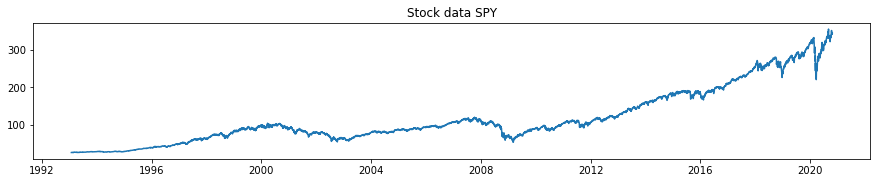(4889, 18) (4889,)
(2096, 18) (2096,)
precision    recall  f1-score   support

0       0.48      0.11      0.18       498
1       0.78      0.96      0.86      1598

micro avg       0.76      0.76      0.76      2096
macro avg       0.63      0.54      0.52      2096
weighted avg       0.71      0.76      0.70      2096

connected to yahoo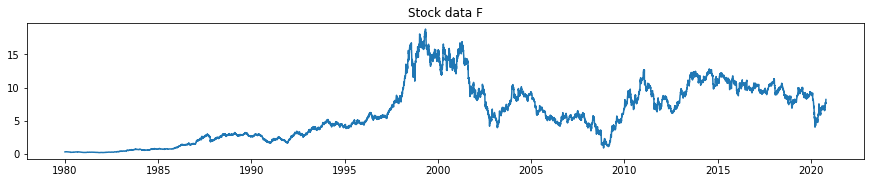(7204, 18) (7204,)
(3088, 18) (3088,)
precision    recall  f1-score   support

0       0.62      0.51      0.56      1318
1       0.68      0.76      0.72      1770

micro avg       0.66      0.66      0.66      3088
macro avg       0.65      0.64      0.64      3088
weighted avg       0.65      0.66      0.65      3088

connected to yahoo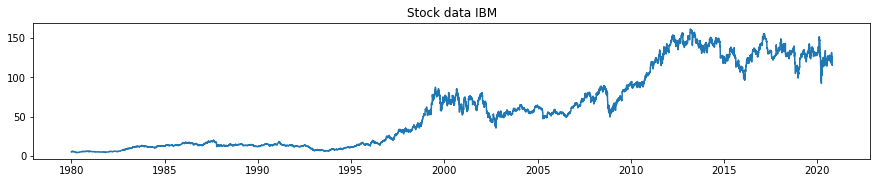(7204, 18) (7204,)
(3088, 18) (3088,)
precision    recall  f1-score   support

0       0.59      0.39      0.47      1202
1       0.68      0.83      0.75      1886

micro avg       0.66      0.66      0.66      3088
macro avg       0.64      0.61      0.61      3088
weighted avg       0.65      0.66      0.64      3088

connected to yahoo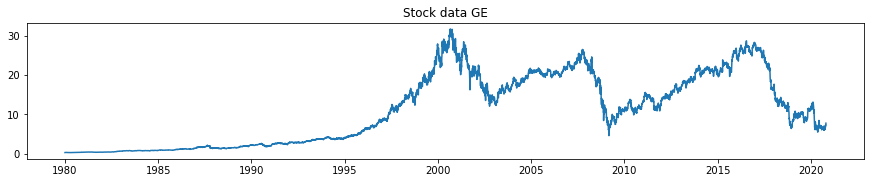(7204, 18) (7204,)
(3088, 18) (3088,)
precision    recall  f1-score   support

0       0.58      0.41      0.48       990
1       0.75      0.86      0.81      2098

micro avg       0.72      0.72      0.72      3088
macro avg       0.67      0.63      0.64      3088
weighted avg       0.70      0.72      0.70      3088

connected to yahoo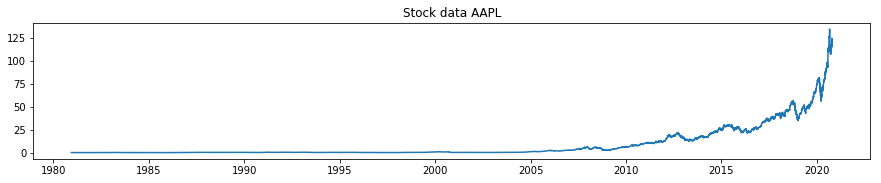(7035, 18) (7035,)
(3016, 18) (3016,)
precision    recall  f1-score   support

0       0.60      0.49      0.54      1087
1       0.74      0.81      0.77      1929

micro avg       0.70      0.70      0.70      3016
macro avg       0.67      0.65      0.66      3016
weighted avg       0.69      0.70      0.69      3016

connected to yahoo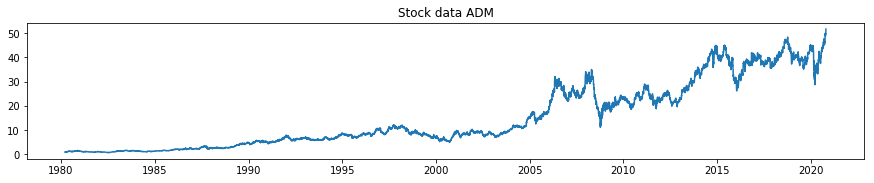(7168, 18) (7168,)
(3072, 18) (3072,)
precision    recall  f1-score   support

0       0.57      0.48      0.52      1198
1       0.70      0.77      0.73      1874

micro avg       0.65      0.65      0.65      3072
macro avg       0.63      0.62      0.63      3072
weighted avg       0.65      0.65      0.65      3072


In [ ]:



## Prediction¶

Here the model will perform trend predictions on an unknown dataset (has not seen it during training or testing).

In :
#ticker='BP'
#ticker='ABBV'
#ticker='GILD'
#ticker='NGG'
ticker='BPY'

In :
# load classifier, no need to initialize the loaded_rf

In :
new_df = get_data(ticker)

connected to yahoo

In :
new_df = compute_technical_indicators(new_df)

In :
new_df = compute_features(new_df)

In :
new_df=define_target_condition(new_df)

In :
new_df = predict_timeseries(new_df, clf)

        Date       High        Low       Open      Close    Volume  Adj Close       EMA5  EMA10  EMA15  ...  aboveEMA100  aboveEMA150  aboveEMA200  aboveUpperBB  belowLowerBB  aboveSAR  oversoldRSI  overboughtRSI  target_cls  Buy
0 2013-03-22  25.000000  21.000000  21.000000  22.030001  314200.0  13.484918   0.000000    0.0    0.0  ...           -1           -1           -1            -1            -1        -1           -1             -1           1    0
1 2013-03-25  22.250000  21.170000  22.090000  21.639999  125000.0  13.246187   0.000000    0.0    0.0  ...           -1           -1           -1            -1            -1        -1           -1             -1           1    0
2 2013-03-26  21.799999  21.129999  21.500000  21.280001  602800.0  13.025826   0.000000    0.0    0.0  ...           -1           -1           -1            -1            -1        -1           -1             -1           1    0
3 2013-03-27  21.959999  21.129999  21.280001  21.360001  628600.0  13.074796   0.000000    0.0    0.0  ...           -1           -1           -1            -1            -1        -1           -1             -1           1    0
4 2013-03-28  21.719999  21.230000  21.389999  21.260000  408700.0  13.013583  13.169062    0.0    0.0  ...           -1           -1           -1            -1            -1        -1           -1             -1           1    0

[5 rows x 47 columns]


Below plots are showing predictions on unseen dataset. When the triangle overlay is on the price data, it means buy. When the triangle is on the zero level, it means, don't buy. This model is pretty much giving only long signals, but cen be extrapolated to sell signals as well.

So the Buy signal means, that the model thinks that in n days (here 55 days) the price will be above specific Exponencial Moving Average (here was trained to be above 150 EMA in 55 days).

We see that the model is giving some false positive signals (of course it is), but not that many actually. It just sometimes expects trend reversal too early, but if we are using this as an investing advisor for long term hold or long term swing trades, the signals provided by the model are very nice.

In :
plot_stock_prediction(new_df, ticker)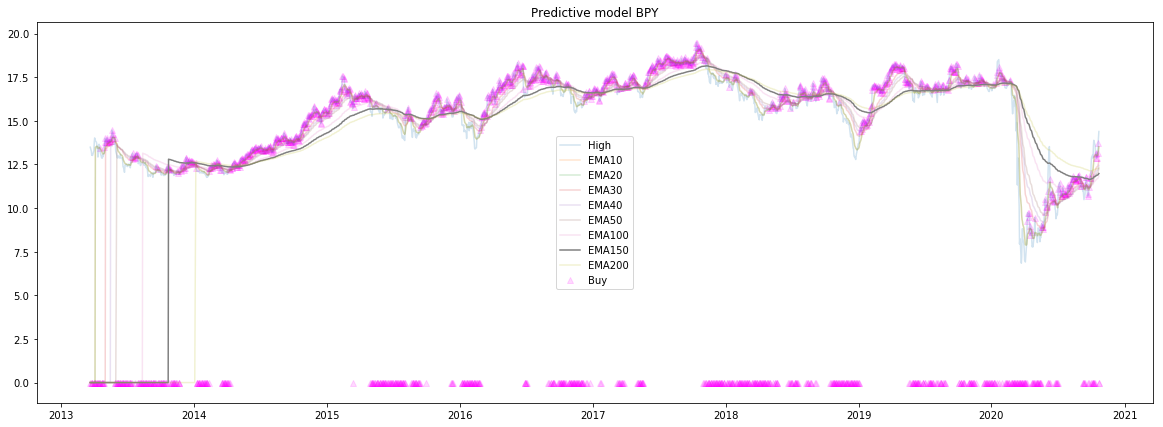In :
# zoom in on the data
temp_df = new_df[-700:]

In :
plot_stock_prediction(temp_df, ticker)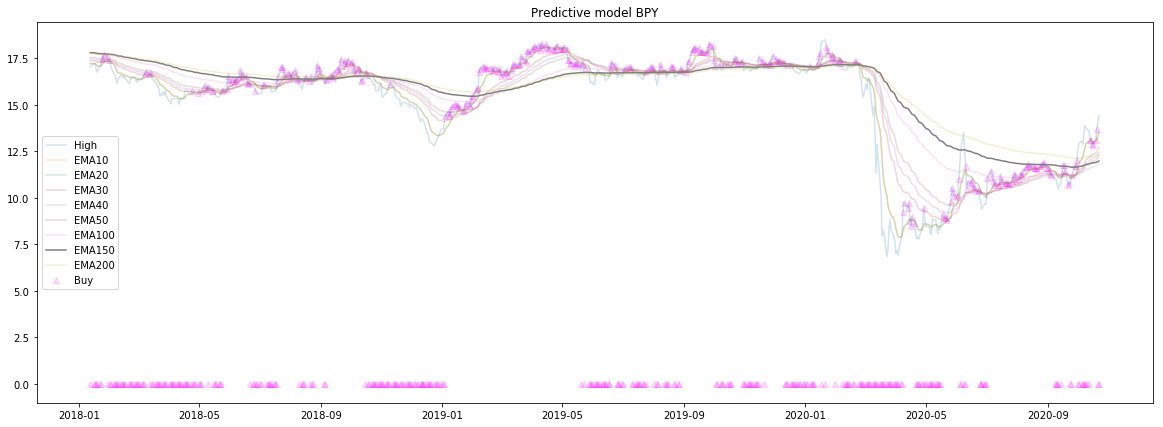#### Summary:¶

Wisdom of the trees (and forests):
Trend is your friend until the end.